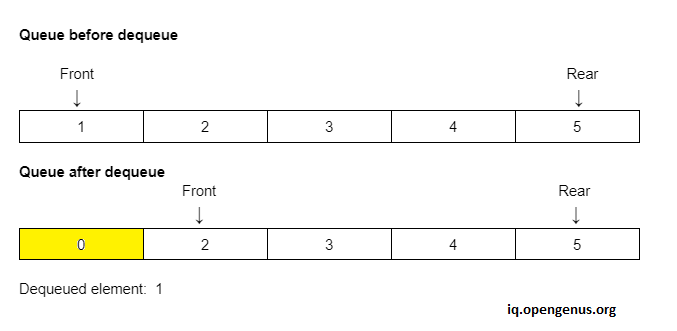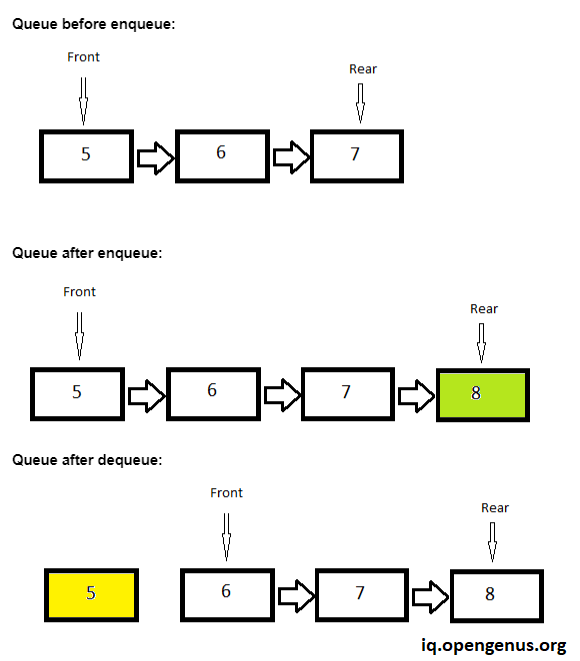×

Search anything:

# Dynamic Queue

#### queue AlgorithmsGet this book -> Problems on Array: For Interviews and Competitive Programming

In this article, we will see what a dynamic queue is and how it is implemented. In short, Dynamic Queue is a Queue whose allocated memory may increase or decrease depending on the number of elements being inserted or deleted in Queue.

1. Introduction to the problem
2. Properties of Queue
3. Queue Operations
4. Queue Implementation with an array
5. Limitations of array usage for queue implementation
6. Dynamic Queue: Queue Implementation with Linked List
7. Comparison of Linked List and Array in the scope of queue implementation
8. Conclusion

### Introduction to problem

In this problem, we are going to see how a dynamic queue i.e. the queue which is resizeable can be implemented.

### Properties of Queue

By definition, a queue is a linear data structure in which insertion and deletion of the elements are performed in such a way that the element which is inserted first will be deleted first from the queue. In other words, the queue data structure works on the principle of FIFO(First In First Out). It can be implemented by using any data structure which behaves in linear fashion i.e. Array, Linked List etc.

### Queue Operations

A typical queue has the following operations.
1 Enqueue
2 Dequeue
Enqueue inserts an element in the queue.
Dequeue removes/deletes an element from the queue.
The elements can only be dequeued from the queue in the same sequence as these are enqueued in the queue.
Enqueue and Dequeue operations typically perform in linear time i.e O(1).

Now, if we talk about the applications of the queue it is the data structure that can be used to implement task scheduling in the CPU etc.

### Queue Implementation with an array

As the queue is a linear data structure, we can implement it using an array because elements store in an array in a linear fashion.

We'll implement it using Java language.

Following is the definition of queue class.

``````class Queue{
private int front;
private int rear;
private int[] queue;

public Queue(int size){
this.queue = new int[size];
this.front = 0;
this.rear = 0;
}

public void enqueue(int value){
// to be implemented
}

public int dequeue(){
// to be implemented
return null;
}

public void printQueue(){
for(int i=0; i < rear; i++){
System.out.println(this.queue[i]);
}
}

}
``````

If we see above there are two pointers being used in the queue class front and rear these would be used for performing insertion and deletion operations in linear time i.e. O(1). Initially, the front and rear both point to the starting element of the queue as we can see 0 value is assigned to both pointers i.e. it refers to the starting index. As the queue performs enqueue/dequeue operations the front and rear pointers get updated accordingly.

Let's see below the implementation of enqueue function and understand how the rear can be used for performing it in linear time.

``````public void enqueue(int value){
if (rear <= queue.length-1){
queue[rear++] = value;
} else {
System.out.println("Queue is full ..");
}
}
``````

Look at the implementation of enqueue function, initially, our rear pointer is at 0 when we enqueue an element it will insert it on the 0th index and increment itself by 1 so that the next time when the element will be inserted it will insert on the next index. This is how the enqueue function put elements in the queue and it will perform this operation in the linear time.

Now let's have look at the implementation of the dequeue function and how front can be used for performing it in linear time.

``````public int dequeue(){
int element = 0;
if (front == rear){
System.out.println("Queue is empty ..");
} else {
element = queue[front];
queue[front] = 0;
front++;
}
return element;
}
``````

Front is pointing to the first element of the queue, as its value is still 0 not manipulated in the enqueue function. When the dequeue function is called the element pointing by the front will be set to 0 refers that the element is removed from the queue and now this space is consumed in the queue then front is incremented by 1 so that now it points to the next element of the queue which will now be the front of the queue.

Following is the complete code of queue with the driver class implemented using an array in java.

``````class Queue{
private int front;
private int rear;
private int[] queue;

public Queue(int size){
this.queue = new int[size];
this.front = 0;
this.rear = 0;
}

public void enqueue(int value){
if (rear <= queue.length-1){
queue[rear++] = value;
} else {
System.out.println("Queue is full ..");
}
}

public int dequeue(){
int element = 0;
if (front == rear){
System.out.println("Queue is empty ..");
} else {
element = queue[front];
queue[front] = 0;
front++;
}
return element;
}

public void printQueue(){
for(int i=0; i < rear; i++){
System.out.println(this.queue[i]);
}
}

}

class Main{
public static void main(String args[]){
Queue queue = new Queue(5);
queue.enqueue(4);
queue.enqueue(5);
queue.enqueue(6);
queue.enqueue(7);
System.out.println("Following are the elements in the queue");
queue.printQueue();
System.out.println("The element dequeued from the queue is " + queue.dequeue());
System.out.println("Following are the elements in the queue");
queue.printQueue();

}
}
``````

### Limitation of array usage for queue implementation

Following are the two major limitations of using an array for the implementation of a queue.

1. Queue Size
2. Memory wastage

#### Queue size:

This is the most common problem one can face when implementing a queue using an array, as array size needs to be declared in advance which is a limitation of array data structure. So, the size of the queue will be limited when it's implemented using an array. As dynamic memory assignment cannot be achieved while using an array, hence a dynamic queue cannot be implemented while using the array.

#### Memory wastage:

You can see from the above implementation when we enqueue an element in the queue we use the rear pointer to perform this operation and when we dequeue an element from the queue we use the front pointer. Element pointing by the front will be retrieved in a variable and this index gets held by the value zero which indicates the element which lies here is removed and front gets incremented to point to the next element in the queue.You can see if an element gets removed from the queue that place of the array (from where the element is removed) is wasted we can't use it again to insert a new element at that position because if we do the working principle of the queue which is FIFO(First In First Out) will be violated.
In this way, much space of array is wasted and will never be able to get used in the future. So, that's how implementing a queue using array results in memory wastage.

## Dynamic Queue: Queue Implementation with Linked List

As Linked List also works in a linear fashion so, we can implement a queue using Linked List.

A Linked List will work as a queue if we insert a new element at the end of the list and removes an element from the top of the list. Similarly, we'll use two pointers front and rear to achieve it as we did in the array.

Following is the complete implementation of queue using Linked List.

``````class Node{
int value;
Node next;

public Node(int value){
this.value = value;
this.next = null;
}

}

class Queue{
private Node front, rear;

public Queue(){
this.front = this.rear = null;
}

public void enqueue(int value){
Node new_node = new Node(value);
if (this.rear == null){
this.front = this.rear = new_node;
} else {
this.rear.next = new_node;
this.rear = new_node;
}
}

public Node dequeue(){
Node element;
if(this.front == null){
element = null;
} else {
element = this.front;
this.front = this.front.next;
element.next = null;
}

if(this.front == null){
this.rear = null;
}

return element;
}

public void printQueue(){
Node node = this.front;
if(node != null){
while(node != null){
System.out.println(node.value);
node = node.next;
}
} else {
System.out.println("Queue is empty ...");
}
}

}

class Main {
public static void main(String[] args) {
Queue queue = new Queue();
queue.enqueue(1);
queue.enqueue(2);
queue.enqueue(3);
queue.enqueue(4);
queue.enqueue(5);
System.out.println("The intial queue is following");
queue.printQueue();
System.out.println("The element which is dequeued: " + queue.dequeue().value);
System.out.println("Now the queue is following");
queue.printQueue();
}
}
``````

From the above implementation it can be seen that when enqueue is called an element is being inserted at the end of Linked List using the rear pointer, the new element gets put in the next of rear and be the new rear, and when dequeue is called an element gets removed from the top of Linked List using front pointer, the element which is being pointed by front gets returned by the dequeue function and front moves 1 position ahead in the Linked List.Both operations are being performed in linear time i.e. O(1).

### Comparison of Linked List and Array in the scope of queue implementation

Ok, let's talk about the comparison of Linked List and Array in this prospect.
As elaborated in the "Limitations of array usage for queue implementation" section to implement a memory-friendly and dynamic queue one cannot use an array because the array size is fixed i.e. needs to be declared in advanced and the queue will never be resized automatically which is the key property of a dynamic queue Moreover, much of the computer memory is wasted while using an array. In contrast of that if a queue is implemented using a linked list it is much more memory efficient and its size can be increased or decreased automatically explained and illustrated in the above section.

### Conslusion

So, all the above discussion concludes that implementing a memory-friendly and dynamic queue Linked List should be the preferred data structure. The array cannot be used to implement a dynamic queue.

One more thing to be noted is that Linked List is better than Array in all prospects while implementing a Dynamic Queue.#### Ali Haider

Currently working as a Software Engineer - WEB in a Fintech company named as i2c Inc. I am continuously striving for best. Completed my BS Computer Science degree from University of Gujrat Pakistan.